# 20. Nonuniformity¶

## 20.1. Introduction to MRI nonuniformity correction¶

FireVoxel’s Nonuniformity tab provides access to several nonuniformity correction methods for MR images. The user may choose the most appropriate method based on the type of images, the severity of nonuniformity artifact and resources available for image processing (such as time and computing power).

Non-uniformity of image intensity is a common MRI artifact. It creates a smooth variation of signal intensity across the image that is unrelated to tissue properties. This artifact may be caused by the imperfections of the imaging technique or the patient’s influence on the magnetic and electric fields. Non-uniformity is often unnoticeable to a human observer, but it may introduce errors into quantitative MRI methods, such as segmentation, registration, and dynamic modeling. To minimize these errors, it is often helpful to remove the non-uniformity artifact before analyzing images.

A number of methods have been developed to minimize the nonuniformity artifacts, including prospective and retrospective methods. Prospective methods rely on additional sequences acquired during the MRI exam and then used during processing for nonuniformity reduction. Retrospective methods, such as the methods available in FireVoxel, estimate the nonuniformities directly, without the need for additional acquisitions. These methods assume that nonuniformity is multiplicative, which means that the intensity of the acquired image at every point can be represented as a product of the corrected intensity and a spatially varying bias field (20.1):

(20.1)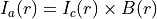whereis the acquired image,is the corrected image andis the bias field. To find the corrected image, we need to estimate the bias field.

## 20.2. Nonuniformity correction methods in FireVoxel¶

FireVoxel offers three retrospective methods for non-uniformity correction: the widely-used N3 method and its variant N4, as well as FireVoxel’s original method, BiCal. The N3 method, for Non-parametric Non-uniformity Normalization (Sled 1998, PMID: 9617910) is offered in the streamlined local implementation (Tsui W, NYU School of Medicine, 2003). The N4 method (Tustison 2010, PMID 20378467), a variant of N3, is included as a plugin, N4BiasFieldCorrection.exe, in the FireVoxel directory. The BiCal method (Mikheev A, Rusinek H, NYU School of Medicine, 2010) is a powerful method for challenging imaging situations, such as abdominal, high field, and accelerated MRI.

The choice of method is usually motivated by a compromise between the quality of correction and computational resources and time available. Among these three methods, N3 is the fastest but the least powerful, and BiCal is the slowest, but may be better suited for complex imaging problems, such as high field imaging.

Additionally, N4 Explorer and BiCal Explorer enable parameter optimization by running corrections with a grid of parameters. The tab also contains options for measuring nonuniformity via coefficient of variation (CV), coefficient of joint variation (CJV), and spillover for two ROI layers.

The correction methods act on 3D or 4D images. For 4D images, if the current image is not the first frame in the dynamic series, FireVoxel shows a warning and asks the user whether to proceed or cancel the correction. If the user chooses to proceed, only the current frame will be corrected. No warning is shown for correction of the first image in dynamic series, and only the first image is corrected.

Each of the three correction commands (N3, N4, and BiCal) opens a dialog panel with adjustable options. The output includes the corrected image or an option to create a bias field map. [N3 – checked checkbox Bias Field creates only bias field, but no corrected image]. The corrected images are placed in new layers named [active_layer]_N3 (or _N4 or _BiCal). Bias field maps are placed in new layers named [active_layer]_N3_BiasField.

## 20.3. N3 and N4 algorithms¶

Below is a brief description of the algorithms for N3 and its variant N4 methods (Fig. 20.1). N3, or Non-parametric Non-uniformity Normalization, is the most commonly used non-uniformity correction method. Both N3 and N4 methods operate in the log-transform space of the image intensities and bias field. These methods deal with the probability densities, or, for discrete images, intensity histograms. The histogram of the logarithm of the bias field is assumed to be a zero-centered Gaussian. Below is an overview of the internal steps of the N3 and N4 algorithms.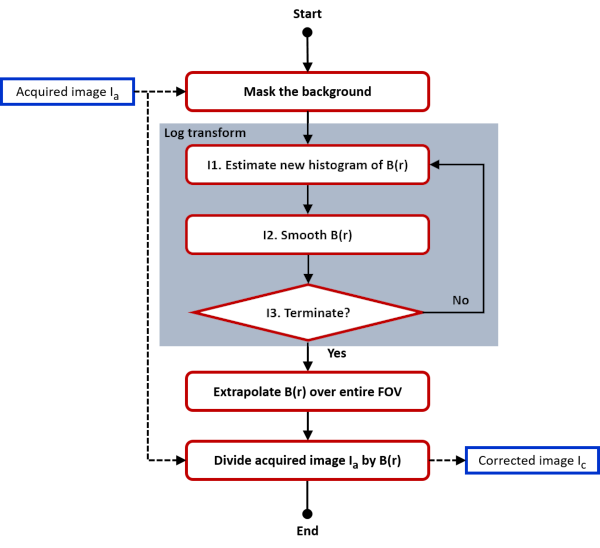Fig. 20.1 N3 or N4 algorithm diagram.

First, the image background is masked. This initial step is followed by an iterative block performed in the log-transform space of the image intensities and bias field. The logarithmic transformation conveniently changes the multiplication to addition, but may run into problems in areas where signal intensity approaches zero, such as the air-filled background regions. Masking the background helps to minimize these potential issues. Within the iterative block, each iteration includes three steps.

In Step 1, the bias field histogram is estimated so that the corrected image is sharpened. This sharpening is achieved by applying the Wiener deconvolution filter to the image intensity histogram. The Wiener filter uses a Gaussian kernel with a full width at half maximum (FWHM) selected by the user.

In Step 2, the new estimate of the bias field is smoothed by fitting it with a three-dimensional B-spline field with a user-specified grid size (given in millimeters). The size of the grid controls the degree of smoothing. This grid size is typically about 200 millimeters for images acquired with a body coil and smaller for images acquired with localized surface coils.

In Step 3, the termination criterion is tested against a specified threshold. In practice, the algorithm stops after a fixed number of iterations specified by the user.

Once the iterations are completed, the bias field is extrapolated over the entire field of view. Finally, the original image is divided by the bias field to obtain the corrected image.

## 20.4. N3 (NYU implementation)¶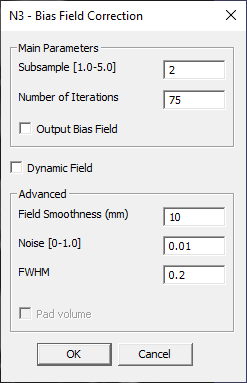Fig. 20.2 N3 parameter panel.

Selecting N3 on the Nonuniformity tab opens a parameter panel (N3 – Bias Field Correction, Fig. 20.2), where the user can specify the method parameters. The three key parameters, as described in the N3/N4 algorithm, are: Number of iterations (exit parameter in Step 3), Field Smoothness (grid size in Step 2), and FWHM of the Gaussian kernel (Step 1).

Dynamic Field (checkbox) – ADD DETAILS

(Pad volume – currently disabled)

By default, the operation creates a corrected image in a newly added layer. Checking Output Bias Field checkbox creates instead a new layer with the bias field map.

## 20.5. N4 (UPenn implementation)¶Fig. 20.3 N4 parameter panel.

The N4 command opens a parameter panel (N4 nonuniformity correction, Fig. 20.3) with parameters of the N3/N4 algorithm: Number of Iterations, Spline Distance (grid size, mm), and FWHM.

Background mask parameters (?):

By default, the output is the corrected image placed in a new layer. Checking Output Bias Field creates instead a new layer with the bias field map.

## 20.6. N4 Explorer¶

Enables optimization of N4 parameters. Requires a visible ROI comprising multiple blobs scattered uniformly across the image. If the document has no visible ROI, the command shows a warning.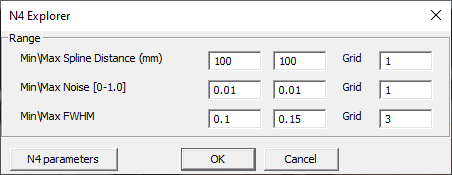Fig. 20.4 N4 Explorer panel.

Opens an N4 Explorer panel (Fig. 20.4) to test combinations of values for three parameters: Spline Distance, Noise, and FVHM. The parameters are sampled on a grid between the minimum and maximum values at a set number of grid steps (Grid).

The results include coefficients aCV, bCV, CSM, blobCJV, voxCJV (Fig. 20.5).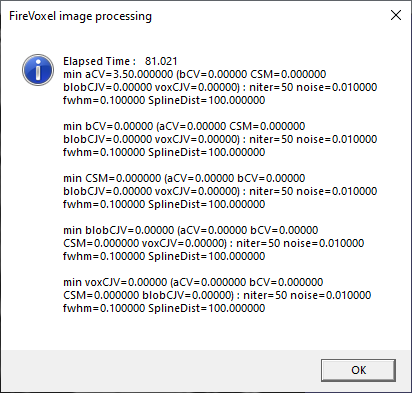Fig. 20.5 N4 Explorer results.

## 20.7. BiCal algorithm¶

BiCal is a powerful and computationally intensive method that may overcome the limitations of the N3/N4 methods in complex imaging tasks.

The BiCal algorithm includes the following main steps. (Fig. 20.6)Fig. 20.6 BiCal algorithm diagram.

First, the background signal is removed from the acquired image to create a background mask. The algorithm then detects sharp edges and creates an edge mask, a key step in this method. The next three action steps are performed in the log-transform space of the image intensities and bias field, as in the N3 and N4 methods.

In the first of these three steps, the partial derivatives of the image intensity are computed for each voxel of the body. The bias field is estimated as a set of smooth polynomial functions and the partial derivatives of the bias field are fitted directly to the partial derivatives of the image signal intensity.

The resulting set of linear equations is solved to obtain the polynomial coefficients that are used to estimate the bias field. Finally, the acquired image is divided by the bias field to obtain the corrected image.

## 20.8. BiCal nonuniformity correction¶

The BiCal command opens a parameter panel for configuring the correction (BiCal Parameters, Fig. 20.7) with the following options.Fig. 20.7 BiCal parameter panel with default values.

Basis functions – Dropdown menu with the choice of polynomial functions used to estimate the bias field:

• Legendre polynomial

• Chebyshev polynomial of the first kind

• Chebyshev polynomial of the second kind

• Regular polynomial

• Cosine.

Processing scale – Sets how many voxels are skipped to speed up processing. Processing scale p indicates that every p-th voxel will be processed.

Field precision (mm) – Sets the degree of polynomial expansion. Related to degree n of basis function expansion, n = image width/field precision.

Max correction – Limits the correction factor:.

Background transition (mm)

Background group – Background (air) region mask parameters:

• Air level (*Noise) (noise=…)

• Low signal level

Region separation – Edge mask parameters:

• Method – Including: Regular Edge, Thin Edge, Gradient (time+)

• Edge Level (noise)

• Region smooth eps

• Adaptive edge (time+)

Output:

(Checkboxes) Real voxels, Work mask, Field (bias field map), All documents

## 20.9. BiCal Explorer¶

Currently unavailable. ADD DETAILS

## 20.10. Measure {CV, CJV, Spillover} for two ROI layers¶

Used to estimate nonuniformity using two distinct tissues. Requires two ROI layers, one in each tissue, with blobs uniformly distributed over the image. Returns basic statistics for each ROI, including number of seeds, average seed intensity, standard deviation, and non-uniformity (Fig. 20.8). Also provides measures derived from matched blobs: contrast average\stdev. ADD DETAILSFig. 20.8 Results of Measure {CV, CJV, Spillover}.

Blob Coefficient of Joint Variation (CJV): Defined from two set of regions, delineated by expert observers in areas of known, uniform tissue throughout the imaged organ (20.2).

(20.2)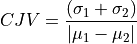whereand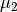are the average values, and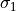and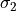are standard deviations of intensity over the two regions. CJV quantifies the intensity variability in each set and controls for the potential undesirable loss of tissue contrast by the algorithm. CJV is quantified before and after correction, a decrease in CJV reflects decreased nonunformity.

Voxel CJV: vxCJV – similar quantity calculated… ADD DETAILS

Optimal Separation misclassification portion… ADD DETAILS and Optimal Threshold… ADD DETAILS

## 20.11. Measure {CJV, Spillover} for two ROI layers (All Documents)¶

Returns Blob CJV, Voxel CJV, and overlap for two ROI layers (Fig. 20.9).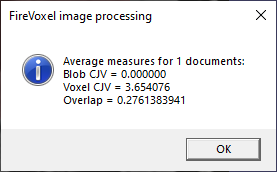Fig. 20.9 Results of Measure {CJV, Spillover}.